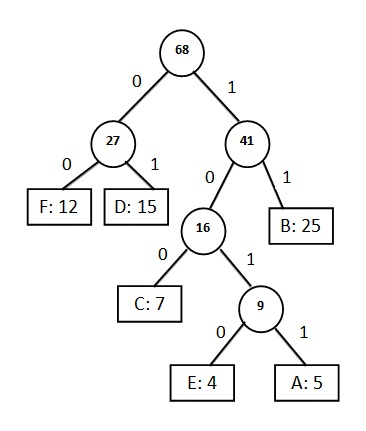# Huffman Coding Algorithm With Example

Huffman coding algorithm was invented by David Huffman in 1952. It is an algorithm which works with integer length codes. A Huffman tree represents Huffman codes for the character that might appear in a text file. Unlike to ASCII or Unicode, Huffman code uses different number of bits to encode letters. If the number of occurrence of any character is more, we use fewer numbers of bits. Huffman coding is a method for the construction of minimum redundancy codes.

Huffman tree can be achieved by using compression technique. Data compression have lot of advantages such as it minimizes cost, time, bandwidth, storage space for transmitting data from one place to another.

In regular text file each character would take up 1 byte (8 bits) i.e. there are 16 characters (including white spaces and punctuations) which normally take up 16 bytes. In the ASCII code there are 256 characters and this leads to the use of 8 bits to represent each character but in any test file we do not have use all 256 characters. For example, in any English language text, generally the character ‘e’ appears more than the character ‘z’. To achieve compression, we can often use a shorter bit string to represent more frequently occurring characters. We do not have to represent all 256 characters, unless they all appear in the document. The data encoding schemes broadly categorized
in two categories.

### Fixed Length Encoding Scheme

Fixed length encoding scheme compresses our data by packing it into the minimum number of bits i.e. needed to represent all possible values of our data. The fixed length code can store maximum 224,000 bits data.

### Variable Length Encoding Scheme

In variable length encoding scheme we map source symbol to variable number of bits. It allows source to be compressed and decompressed with zero error.

### Construction of Huffman Code

A greedy algorithm constructs an optimal prefix code called Huffman code. The algorithm builds the tree T corresponding to the optimal code in a bottom-up manner. It begins with a set of |C| leaves (C is the number of
characters) and perform |C| – 1 ‘merging’ operations to create the final tree. In the Huffman algorithm ‘n’ denotes the number of set of characters, z denotes the parent node and x & y are the left & right child of z respectively.

Huffman (C)
1. n=|C|
2. Q=C
3. for i=1 to n-1
4. do
5. z=allocate_Node()
6. x=left[z]=Extract_Min(Q)
7. y=right[z]=Extract_Min(Q)
8. f[z]=f[x]+f[y]
9. Insert(Q,z)
10. return Extract_Min(Q)

#### Analysis

The Q is initialized as a priority queue with the character C.
Q=C can be performed by using Build_Heap in O(n) time.
For loop takes (|n|-1) times because each heap operation requires O(log n) time.
Hence the total running time of Huffman code on the set of n characters is O(n log n).

#### Method

The following general procedure is applied for construction a Huffman tree:
Search for the two nodes having the lowest frequency, which are not yet assigned to a parent node.
Couple these nodes together to a new interior node.
Add both the frequencies and assign this value to the new interior node.
The procedure has to be repeated until all nodes are combined together in a root node.

## Huffman Coding Algorithm Example

Construct a Huffman tree by using these nodes.

 Value A B C D E F Frequency 5 25 7 15 4 12

Solution:

Step 1: According to the Huffman coding we arrange all the elements (values) in ascending order of the frequencies.

 Value E A C F D B Frequency 4 5 7 12 15 25

Step 2: Insert first two elements which have smaller frequency.Value C EA F D B Frequency 7 9 12 15 25

Step 3: Taking next smaller number and insert it at correct place.Value F D CEA B Frequency 12 15 16 25

Step 4: Next elements are F and D so we construct another subtree for F and D.Value CEA B FD Frequency 16 25 27

Step 5: Taking next value having smaller frequency then add it with CEA and insert it at correct place.Value FD CEAB Frequency 27 41

Step 6: We have only two values hence we can combined by adding them.Huffman Tree
 Value FDCEAB Frequency 68

Now the list contains only one element i.e. FDCEAB having frequency 68 and this element (value) becomes the root of the Huffman tree.

If you found anything missing or incorrect in above huffman coding tutorial then please mention it by commenting below.

### 12 thoughts on “Huffman Coding Algorithm With Example”

1.What shall we do if we have the same rezult for examle EA=9 and C=9, how to align them??

1.in that case c/9 and f/12 form a subtree…

2.please keep the program for huffman algorithm

1.3.assignment code

i love to study data structure

4.This was very verymuch helpful ty so much…..4 sharing this

1.What if we place the alphabets having higher frequency in the left and lower at the right? The solution given above provides a longer code for B than F (in decimal) which goes against the huffman rule. But as per the suggestion the vulnerability can be removed.. Correct me if I am wrong

5.I need Huffman coding program using matab

6.What’s the running time of that algorithm

7.That was great tutorial on Huffman tree, i searched many other ones but this is the best. Keep it up sir

8.If the variable length code (Huffman code) is given for all the characters. Plus their frequency is also given except for one character. Now how to find the missing frequency range of that character?

9.best explanation I found….. thank you